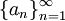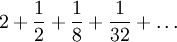# Introduction to Infinite Series

Previous: Infinite Series

Next: Convergence of Infinite Series

Suppose that we are given an infinite sequenceand that we would like to add its elements togetherwhich would produce an infinite series or an infinite sum, as was introduced in the previous lesson. Using sigma notation, we can write this sum asThis simple construction leads us to two immediate questions:

1. How do we know if the infinite sum produces a finite number?
2. If the given infinite sum does yield a finite number, what is it?

These two questions and their answers are the subject of this lesson and a key component of this module on sequences and infinite series.

## Example

Consider the following example. Suppose we would like to determine the sum of the infinite seriesIndeed, we are faced with the two questions listed above: does this sequence yield a finite number, and if it does, what is it? Given a general problem, the answers to these two questions are not straightforward. In this lesson, we will introduce one approach to answer these two questions if the given sum has a special form. We will see that because the infinite sequence above has this particular form, we can calculate its sum quite easily.

Previous: Infinite Series

Next: Convergence of Infinite Series